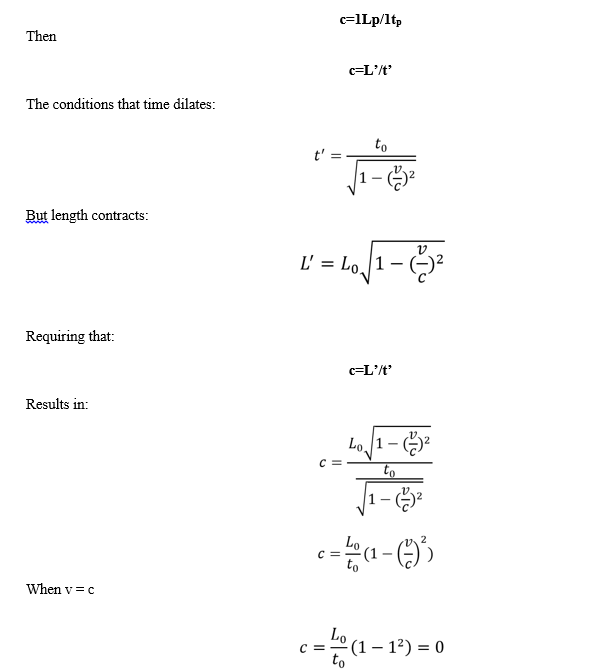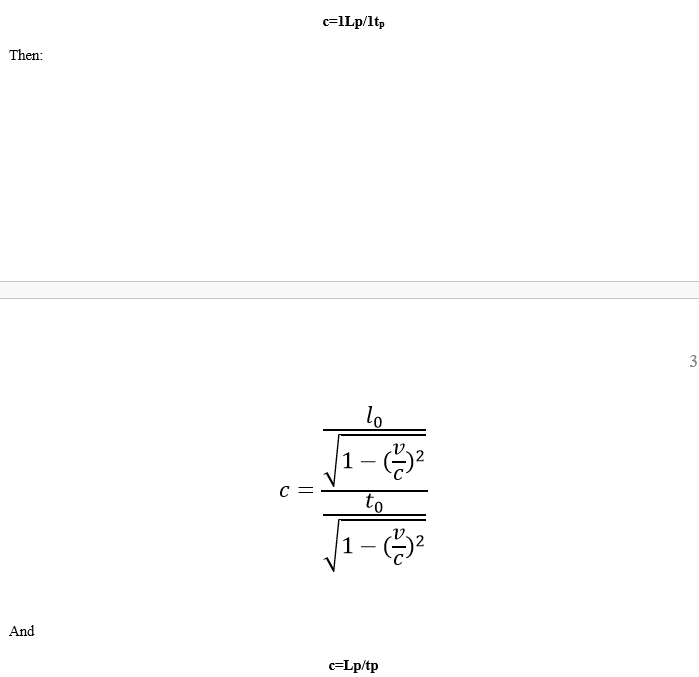wbilly3814

New Members

1

Posts posted by wbilly3814

The meter-second (moved from general discussion)

The G x h-bar does not result in m*s, it result in s, even in GR by setting G/c^4 to 1 results in m*s^2/Kg

the meter*second is a failed use of the derivation of 'length contraction,' where invariably the confused scientists cone to a point in the derivation such that L x T = l' x t'

Thus they inadvertently can get to L/t'=l'/T and it's all downhill from there.

The problem is, if you use length contraction, l't' is always equal to 1.  So the reason they are able to derive the bizarre inept result of length contraction is because they have '1' on the other side of the equal sign, which allows them to commit all kinds of tom foolery.

Length dilates, just as time dilates:That is, if length contracts and time dilates, at v=c, c=0. In fact, c is variable across the spectrum.

However if we dilate length:And the c is preserved and constant.

dr wjb

×
×
• Create New...